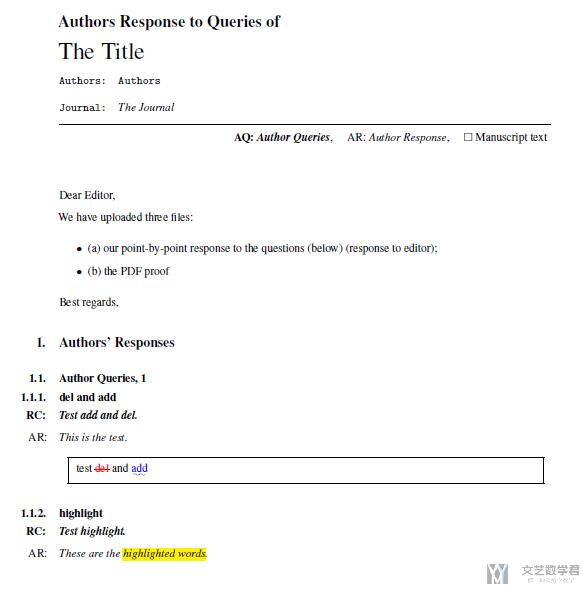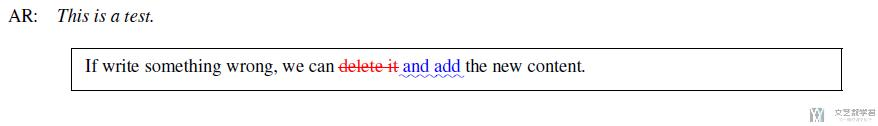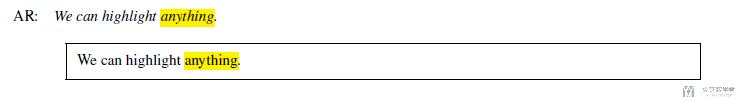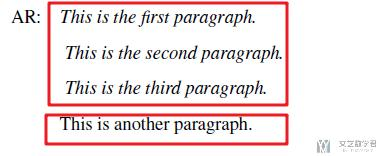# Latex模版–Review and Response Letters2020年4月21日07:55:58

4 2951字阅读9分50秒

## 模型的简单使用

### 一个最简单的模板

1. \documentclass{article}
2.     \def\papertitle{The Title}
3.     \def\authors{Authors}
4.     \def\journal{The Journal}
5. \input{../templates/AR_to_RC.tex}
6. % ----------
8. % ----------
9. ~\newline
10. Dear Editor,
11. We have uploaded three files: % 这次上传的三个文件包括
12. \begin{itemize}
13.     \item (a) our point-by-point response to the questions (below) (response to editor);
14.     \item (b) the PDF proof
15. \end{itemize}
16. Best regards,
17. % -----------
18. % Reviewer 1
19. % -----------
20. \section{Authors' Responses}
21. % 第一个问题
22. \subsection{Author Queries, 1}
23. % 1.1
25. \RC Test add and del.
26. \AR This is the test.
27. \begin{quote}
28.     \DIFdelbegin
32.     \DIFdelend
33. \end{quote}
34. % 1.2
35. \subsubsection{highlight}
36. \RC Test highlight.
37. \AR These are the \hl{highlighted words}.
38. \end{document}### 文字删除和新增高亮

1. \AR This is a test.
2. \begin{quote}
3.     \DIFdelbegin
5.         If write something wrong, we can \DIFdel{delete it} \DIFadd{and add} the new content.
7.     \DIFdelend
8. \end{quote}### 黄色高亮

1. \AR We can highlight \hl{anything}.
2. \begin{quote}
3.     We can highlight \hl{anything}.
4. \end{quote}### 多段文字的处理

1. \AR This is the first paragraph.
2. \AR* This is the second paragraph.
3. \AR* This is the third paragraph.
4. This is another paragraph.### 模版的修改

1. % Define title defaults if not defined by user (自定义标题)
2. \providecommand{\lettertitle}{Authors Response to Queries of}
3. \providecommand{\papertitle}{Title}
4. \providecommand{\authors}{Authors}
5. \providecommand{\journal}{Journal}
6. \input{../templates/preamble.tex}
7. \begin{document}
8. % Make title (需要在这里指定需要出现的内容)
9. {\Large\bf \lettertitle}\\[1em]
10. {\huge \papertitle}\\[1em]
11. \texttt{\textbf{Authors:} \authors}\\
12. \\
13. \texttt{\textbf{Journal:} \it \journal}\\
14. \hrule
15. % Legend(注释AR表示什么意思)
16. \hfill {\bfseries AQ:} \textbf{\textit{Author Queries}},$$\quad$$ AR: \emph{Author Response}, $$\quad\square$$ Manuscript text

1. \usepackage{suffix}
2. % 原始的RC的设置
3. \long\def\RC#1\par{\makebox[0pt][r]{\bf RC:\hspace{4mm}}\textbf{\textit{#1}}\par} %\RC
4. \WithSuffix\long\def\RC*#1\par{\textbf{\textit{#1}}\par} %\RC*
5. % 新增的AQ的设置
6. \long\def\AQ#1\par{\makebox[0pt][r]{\bf AQ:\hspace{4mm}}\textbf{\textit{#1}}\par} %\AQ
7. \WithSuffix\long\def\AQ*#1\par{\textbf{\textit{#1}}\par} %\AQ*

• 微信公众号
• 关注微信公众号
•• QQ群
• 我们的QQ群号
•• 本文由 发表于 2020年4月21日07:55:58
• 转载请务必保留本文链接：https://mathpretty.com/12109.html